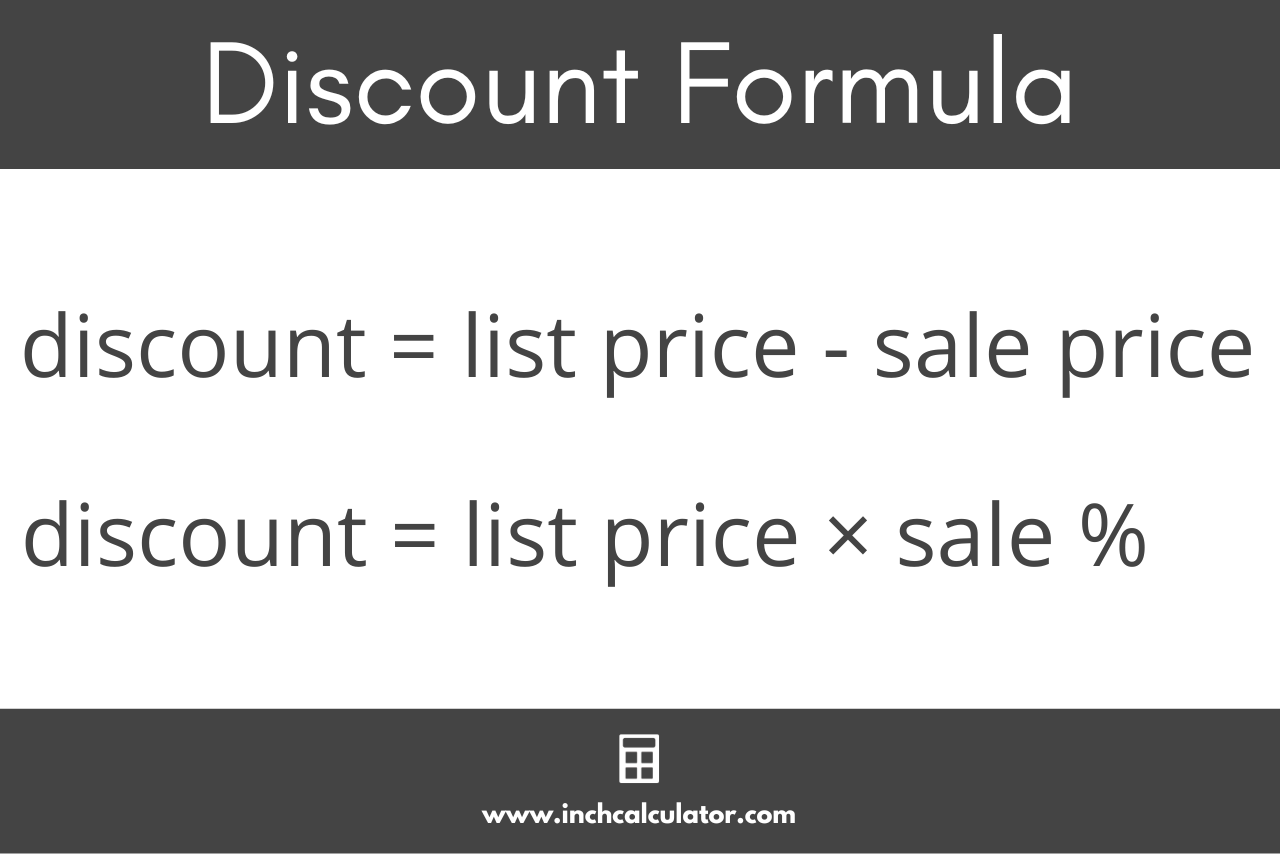# Discount Calculator

Enter the initial price and either the fixed discount or percentage off to calculate the final price of an item and see how much money you’ll save.

\$
\$

\$
%

## Price Results:

final price

percent off
Learn how we calculated this below

## How to Calculate a Discount

A discount is a price reduction or decrease from the original price of an item or service. Think of it as the amount of money you’ll save by purchasing an item at a lower price compared to purchasing it at its regular price.

Whether it’s for a Black Friday or Cyber Monday sale, you can calculate the discount given the original price and the final sale price using a formula.

discount = original price – sale price

Thus, the discount amount is equal to the original price minus the sale price.For example, let’s calculate the discount on an item that is normally \$50 but is on sale for \$40.

discount = \$50 – \$40 = \$10

Thus, the sale price is a \$10 discount.

## How to Convert a Discount to a Percentage

A discount might seem like a bargain, but it’s all relative to the original price. A \$5 reduced price for a \$1,000 item is not nearly as significant as it is for a \$10 item, even though the dollar amount of the discount is the same.

You can find the sale percentage if you know the initial price and the discount amount in a few simple steps.

First, divide the discount by the initial price to get the sale percentage in decimal form.

Then, multiply by 100 to get the discount percentage.

For example, let’s calculate the discount percentage of an item that is \$25 with a \$5 off sale.

Start by finding the sale percentage as a decimal.

decimal percent = \$5 ÷ \$25 = 0.2

Then multiply by 100 to get the discount as a percent.

discount percentage = 0.2 × 100 = 20%

So, the result is a 20% discount.

Here’s a tip: use our decimal to percent calculator to help convert it to a percentage.

## How to Calculate the Sale Price

For fixed-amount discount sales, you can calculate the final sale price of an item after the discount. Simply subtract the fixed discount from the initial price to get the sale price of an item.

sale price = original price – fixed discount

For example, let’s calculate the sale price of a \$25 item that is on sale for \$5 off.

sale price = \$25 – \$5 = \$20

So, the sale price after the discount is \$20.

### How to Calculate a Percent Off Sale Price

Discounts are sometimes advertised as a percentage off of the original price. To calculate the sale price of an item if you know the discount percentage, follow a few simple steps.

First, convert the discount percentage to a decimal number by dividing by 100 (or use a calculator).

Then, multiply the decimal value by the price to find the discount amount.

Finally, subtract the discount amount from the initial price to find the final sale price.

For example, let’s find the sale price of an item that is \$20 with a 25% discount.

Convert the sale percentage to a decimal:

decimal percent = 25% ÷ 100 = 0.25

Find the discount amount:

discount = \$20 × 0.25 = \$5

Find the sale price:

sale price = \$20 – \$5 = \$15

So, the sale price after the discount is \$15. You can also use our percent off calculator to calculate the discount in dollars of a percentage off.

### How to Calculate a Quantity Discount

Some merchants and suppliers offer discounts for the purchase of a quantity of more than one; this is referred to as a quantity discount. For instance, a vendor may sell an item for \$5 if you buy one, or \$40 for 10.

To calculate a quantity discount, divide the sale price by the number of items to find the price per item. Then, use the formula above to calculate the discount by subtracting the price per item in the bundle from the original price for a single item.

For example, let’s find the price of an item that is \$5 if you buy one, or \$40 for 10.

price per item = \$40 ÷ 10 items = \$4 per item
discount = \$5 original price – \$4 discounted price = \$1
decimal percent = \$1 ÷ \$5 = 0.2
discount percentage = 0.2 × 100% = 20%

Therefore, the quantity discount of the item is 20% off per item when you buy 10.

## Sale Prices After Common Discounts

Table showing the final price after a fixed discount off of the initial price.
Initial Price Price After Discount
\$5 off \$10 off \$15 off \$20 off \$25 off
\$50.00 \$45.00 \$40.00 \$35.00 \$30.00 \$25.00
\$60.00 \$55.00 \$50.00 \$45.00 \$40.00 \$35.00
\$70.00 \$65.00 \$60.00 \$55.00 \$50.00 \$45.00
\$80.00 \$75.00 \$70.00 \$65.00 \$60.00 \$55.00
\$90.00 \$85.00 \$80.00 \$75.00 \$70.00 \$65.00
\$100.00 \$95.00 \$90.00 \$85.00 \$80.00 \$75.00
\$110.00 \$105.00 \$100.00 \$95.00 \$90.00 \$85.00
\$120.00 \$115.00 \$110.00 \$105.00 \$100.00 \$95.00
\$130.00 \$125.00 \$120.00 \$115.00 \$110.00 \$105.00
\$140.00 \$135.00 \$130.00 \$125.00 \$120.00 \$115.00
\$150.00 \$145.00 \$140.00 \$135.00 \$130.00 \$125.00
\$160.00 \$155.00 \$150.00 \$145.00 \$140.00 \$135.00
\$170.00 \$165.00 \$160.00 \$155.00 \$150.00 \$145.00
\$180.00 \$175.00 \$170.00 \$165.00 \$160.00 \$155.00
\$190.00 \$185.00 \$180.00 \$175.00 \$170.00 \$165.00
\$200.00 \$195.00 \$190.00 \$185.00 \$180.00 \$175.00